## Other important rules

Important relations that follow from the basic rules are (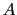is also a generic hypothesis):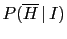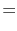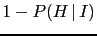(29)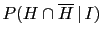0 (30)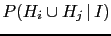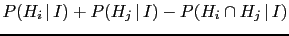(31)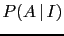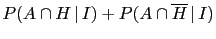(32)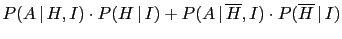(33)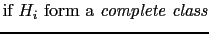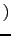(34)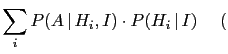idem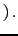(35)

The first two rules are quite obvious. Eq. (31) is an extension of the third basic rule in the case two hypotheses are not mutually exclusive. In fact, if this is not case, the probability of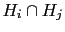is double counted and needs to be subtracted. Eq. (32) is also very intuitive, because eitheris true together with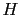or with its opposite.

Formally, Eq. (33) follows from Eq. (32) and basic rule 4. Its interpretation is that the probability of any hypothesis can be seen as weighted average' of conditional probabilities, with weights given by the probabilities of the conditionands [remember that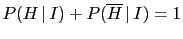and therefore Eq. (33) can be rewritten as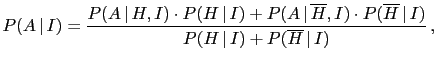that makes self evident its weighted average interpretation].

Eq. (34) and (35) are simple extensions of Eq. (32) and (33) to a generic complete class', defined as a set of mutually exclusive hypotheses [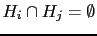, i.e.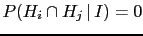], of which at least one must be true [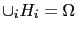, i.e.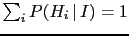]. It follows then that Eq. (35) can be rewritten as the (`more explicit') weighted average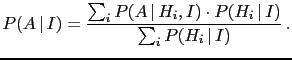[Note that any hypothesisand its opposite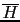form a complete class, because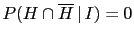and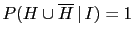.]
Giulio D'Agostini 2010-09-30